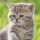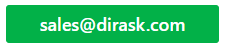Home
IT Knowledge
IT Career
Inspiration
Languages
EN

# JavaScript - How to use let with code example?

1 points
Created by:Kate_C
19800

`let` keyword has been introduces to JavaScript in ES2015. There are few importantn things that should know each programmer who want to use `let` keyword.

## 1. `let` keyword syntax example

`let` syntax is simillar to `var.`

1. varialbe declaration
``````let a;
let b;
let c;``````
2. variable declaration with value assigning
``````let a = 1;
let b = 2;
let c = 3;``````
3. if variable is not assigned
``````let a;
let b;

console.log(a === undefined); // true
console.log(b === udeffined); // true``````

Output:

``````true
true``````
4. multiple variables declaration with one `let` keyword
``````let a, b, c;

console.log(a === undefined); // true
console.log(b === undefined); // true
console.log(c === undefined); // true``````

Output:

``````true
true
true``````
5. multiple variables declaration with value assigning
``````let a = 1, b = 2, c = 3;

console.log(a); // 1
console.log(b); // 2
console.log(c); // 3``````

Output:

``````1
2
3``````

6. multiple variables declaration, some with value assigning

``````let a, b = 2, c;

console.log(a); // undefined
console.log(b); // 2
console.log(c); // undefined``````

Output:

``````undefined
2
undefined``````

## 2. `let` keyword scope example

The scope of `let` is the nearest enclosing curly brackets.

``````let a = 1;

{ // this brackets create new scope for let keyword variables
let a = 2;

console.log(a); // 2
}

console.log(a); // 1``````

Output:

``````2
1``````

## 3. `let` keyword with `for` loop

When `let` keyword is used with `for` loop the scope of varilabe is for whole `for` statement.

``````for (let i = 0; i < 5; ++i) {
console.log(i);
}

// i variable cannot be used here
// var keyword allowed to use variables here``````

Output:

``````0
1
2
3
4``````

## 4. Difference between `let` and `var` keywords

Difference has been described here.

## 5. Tips

• ALWAYS use `let` keyword.

## Merged questions

1. How to use let in JavaScript?
2. JavaScript - How to use let?